Courses

# Test: Shear Force & Bending Moment - 1

## 10 Questions MCQ Test Topicwise Question Bank for Mechanical Engineering | Test: Shear Force & Bending Moment - 1

Description
This mock test of Test: Shear Force & Bending Moment - 1 for Mechanical Engineering helps you for every Mechanical Engineering entrance exam. This contains 10 Multiple Choice Questions for Mechanical Engineering Test: Shear Force & Bending Moment - 1 (mcq) to study with solutions a complete question bank. The solved questions answers in this Test: Shear Force & Bending Moment - 1 quiz give you a good mix of easy questions and tough questions. Mechanical Engineering students definitely take this Test: Shear Force & Bending Moment - 1 exercise for a better result in the exam. You can find other Test: Shear Force & Bending Moment - 1 extra questions, long questions & short questions for Mechanical Engineering on EduRev as well by searching above.
QUESTION: 1

### If the section modulus of a beam decreases, then bending stress will

Solution: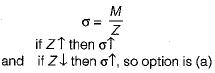QUESTION: 2

### For a given stress, the ratio of moment of resistance of a square beam with its sides horizontal, compared to when the diagonal is horizontal is

Solution: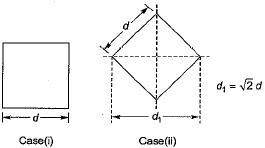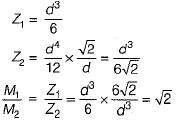QUESTION: 3

### The moment diagram for a cantilever beam subjected to bending moment at end of beam will be

Solution: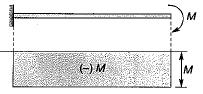QUESTION: 4

If the load at free end on a cantilever beam is increased so as to cause rupture, same will occur

Solution:

Reaction generated at fixed end would be same as end applied load.

QUESTION: 5

Shear force at any point on the beam is the algebraic sum of

Solution:
QUESTION: 6

Bending moment at any point is equal to the algebraic sum of

Solution:
QUESTION: 7

The rate of change of shearing force at any section is equal to the rate of

Solution:
QUESTION: 8

The reaction (in kN) at the support 'A' for the beam shown in the given figure is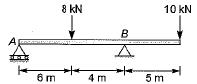Solution: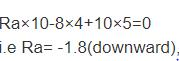QUESTION: 9

A beam carrying a uniformly distributed load rests on two supports ‘b’ apart with equal overhangs ‘a’ at each end. The ratio b/a for zero bending moment at mid-span is

Solution: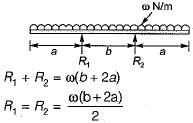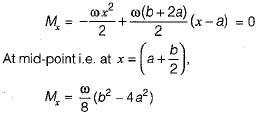This point is also the point of contra-fiexure and it will be zero at b = 2a.
So, answer is (d).

QUESTION: 10

The point of contra-flexure occurs only in

Solution: